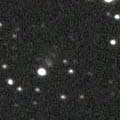# \$B%K!<%H(B-\$B%j%K%"WB@1(B

P/2002 T6 ( NEAT-LINEAR )###\$B%W%m%U%#!<%k(B

 \$BH/8+F|(B 2002\$BG/(B10\$B7n(B4\$BF|(B, 10\$B7n(B7\$BF|(B \$BH/8+8wEY(B 20.1\$BEy(B, 19.4\$BEy(B \$BH/8+ Near-Earth-Asteroid Tracking Team (Jet Propulsion Laboratory), Lincoln Laboratory Near-Earth Asteroid Research project

###\$B###\$B50F;MWAG(B

```                    Epoch = 2003 June 10.0 TT
T = 2003 June 27.01545 TT        Peri. = 217.57304
e = 0.5573311                    Node  = 209.04572 2000.0
q = 3.3870835 AU                 Incl. =  11.00925
a =  7.6515059 AU   n = 0.04656758   P =  21.16 years
```

###\$B@1?^(B###\$B8wEYJQ2=(B

```        m1 = -7 + 5 log\$B&\$(B + 38.5 log r(t - 117)
```##### \$B50F;MWAG\$O!"(BCBET 5306\$B\$K7G:\\$5\$l\$?\$b\$N\$G\$9!#(B \$B@1?^\$O(B StellaNavigator Ver.2.0 for Windows (\$B%"%9%H%m%"!<%D(B \$BJTCx(B / \$B%"%9%-!<=PHG6I4)(B) \$B\$G:n@.\$7\$?\$b\$N\$G\$9!#(B \$B8wEY%0%i%U\$O(BComet for Windows\$B\$G:n@.\$7\$?\$b\$N\$G\$9!#(B